Fall 2003

# Mathematics Math21b Fall 2003

## Linear Algebra and Differential Equations

Office: SciCtr 434
Email: knill@math.harvard.edu# The Good Will Hunting Problem

(A problem in Linear Algebra)
 In the movie "Good Will Hunting", the main character Will Hunting (Matt Damon) solves a blackboard problem, which had been assigned as a challenge to a linear algebra class. [ Update: 2013: Non flash video Update: Jan 2017: A larger size clip with the math only.] Update: May 2017, Thanks to Mark W. Wiemer for some corrections to 3) and 4) which are here applied.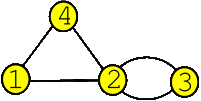1) Find the adjacency matrix A of the graph G 2) Find the matrix giving the number of 3 step walks in G. 3) Find the generating function for walks from point i to j. 4) Find the generating function for walks from points 1 to 3.
The adjacency matrix L encodes the graph. The entry Lij is equal to k if there are k connections between node i and j. Otherwise, the entry is zero. Problem 2 asks to find the matrix which encodes all possible paths of length 3. Generating function. To a graph one can assign for pair of nodes i,j a series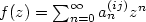, where an(ij) is the number of walks from i to j with n steps. Problem 3) asks for a formula for f(z) and in problem 4) an explicit expression in the case i=1,j=3.

 Solution to 1). The adjacency matrix L is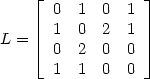Solution to 2). [L2]ij is by definition of the matrix product the sum Li 1 L1 j + Li 2 L2 j +... + Li n Ln j. Each term Li k Lk j is not 0 if and only if there is at least one path of length 2 going from i to j passing through k. Therefore [L2]ij is the number of paths of length 2 leading from node i to j. Similarly, [Ln]ij is the number of paths of length n going from i to j. The answer is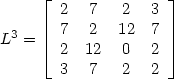Solution to 3). The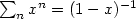for the summation of a geometric series holds also for matrices: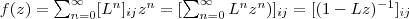. Cramer's rule for the inverse of a matrix is A-1 = adj(A)/det(A) where adj(A) is the adjugate matrix of A, the transpose of the cofactor matrix of A. Therefore, we have for every i and j the generating function adj(1-z L)ij/det(1-z L) which is a rational function. Solution to 4). Especially, when i=1 and j=3, we get adj(1-zL)13 = 2 z2 + 2 z3 and det(1-zL) = 1-7 z2 - 2 z3+4 z4. The result can be written as (2 z2+2z3)/(1-7 z2 - 2 z3+4 z4) which simplifies to 2z^2/(1-z-6z^2+4z^3). Handout [PDF]

 Here is Mathematica code to check this: L={{0,1,0,1}, {1,0,2,1}, {0,2,0,0}, {1,1,0,0}} K[z_]:=IdentityMatrix -z*L; Adj[A_,i_,j_]:=Module[{B=A},B=Delete[B,i];B=Transpose[B];B=Delete[B,j];B]; (-1)^(1+3) Det[Adj[K[z],1,3]]/Det[K[z]] (* check with Inverse[K[z]][[1,3]] *) 

Back to the main page